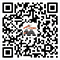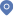首页 - 科研队伍• 研究方向：

随机波动方程反问题，随机偏微分方程数值计算
• 代表作：

```1. (with J. Li and P. Li) Inverse elastic scattering for a random potential, SIAM J. Math. Anal., 54 (2022), 5126–5159.
2. (with P. Li) An inverse random source problem for Maxwell’s equations, Multiscale Model. Simul., 19 (2021), 25–45.
3. (with C.-E. Bréhier) On parareal algorithms for semilinear parabolic stochastic PDEs, SIAM J. Numer. Anal., 58 (2020), 254–278.
4. (with J. Hong) Invariant Measures for Stochastic Nonlinear Schrödinger Equations, Lecture Notes in Mathematics 2251, Springer, Singapore, 2019.
5. (with J. Hong and L. Zhang) Numerical analysis on ergodic limit of approximations for stochastic NLS equation via multi-symplectic scheme, SIAM J. Numer. Anal., 55 (2017), 305–327.```
• 联系方式：

办公室：中科院数学院科技综合楼520房间 电　话：0086-10-82544410 邮　箱： wangxu@lsec.cc.ac.cn